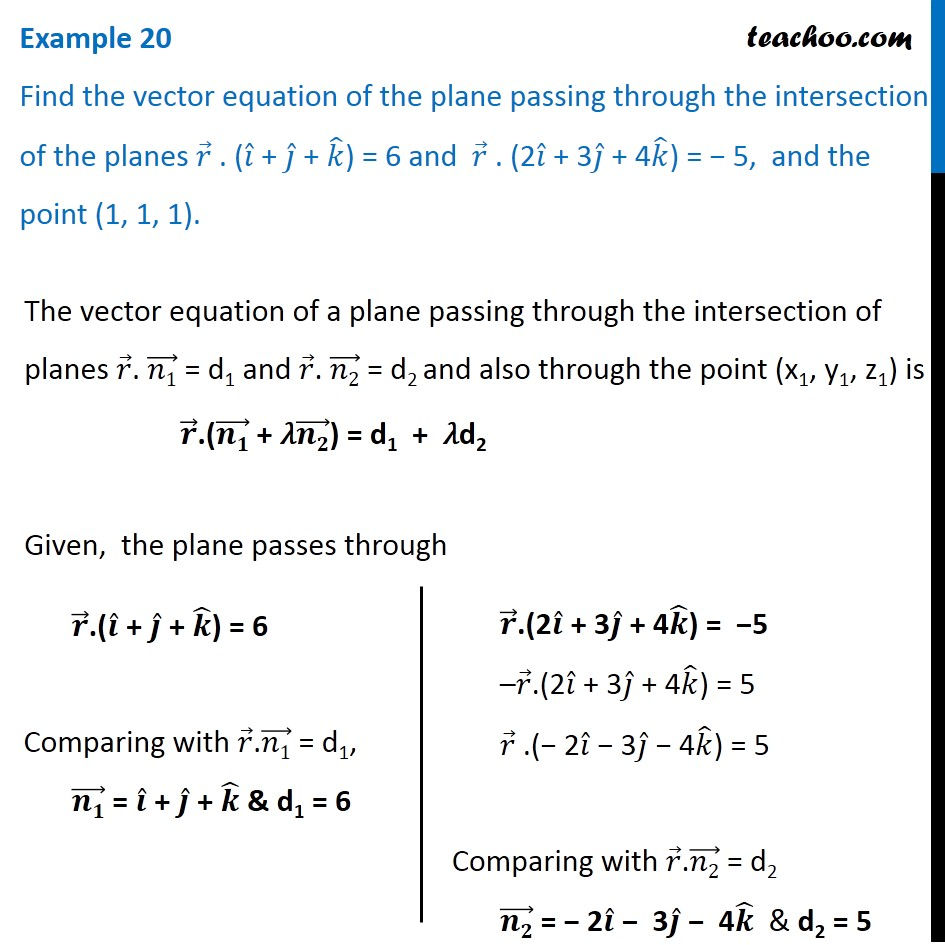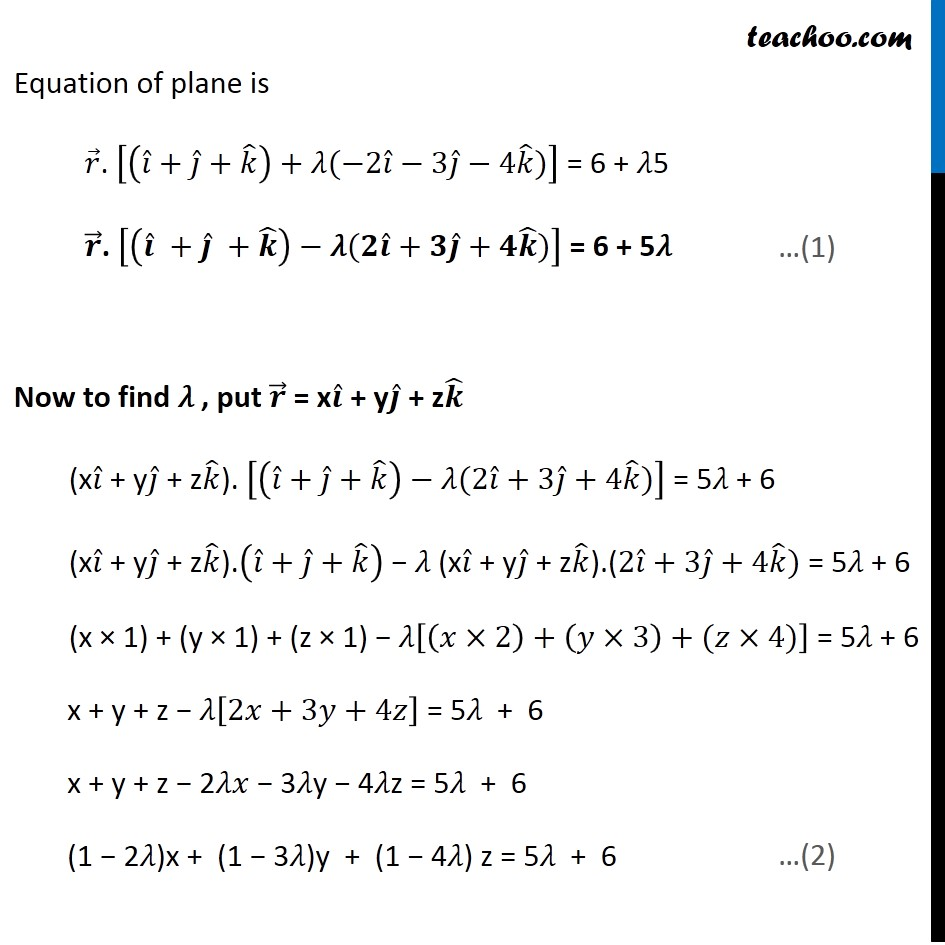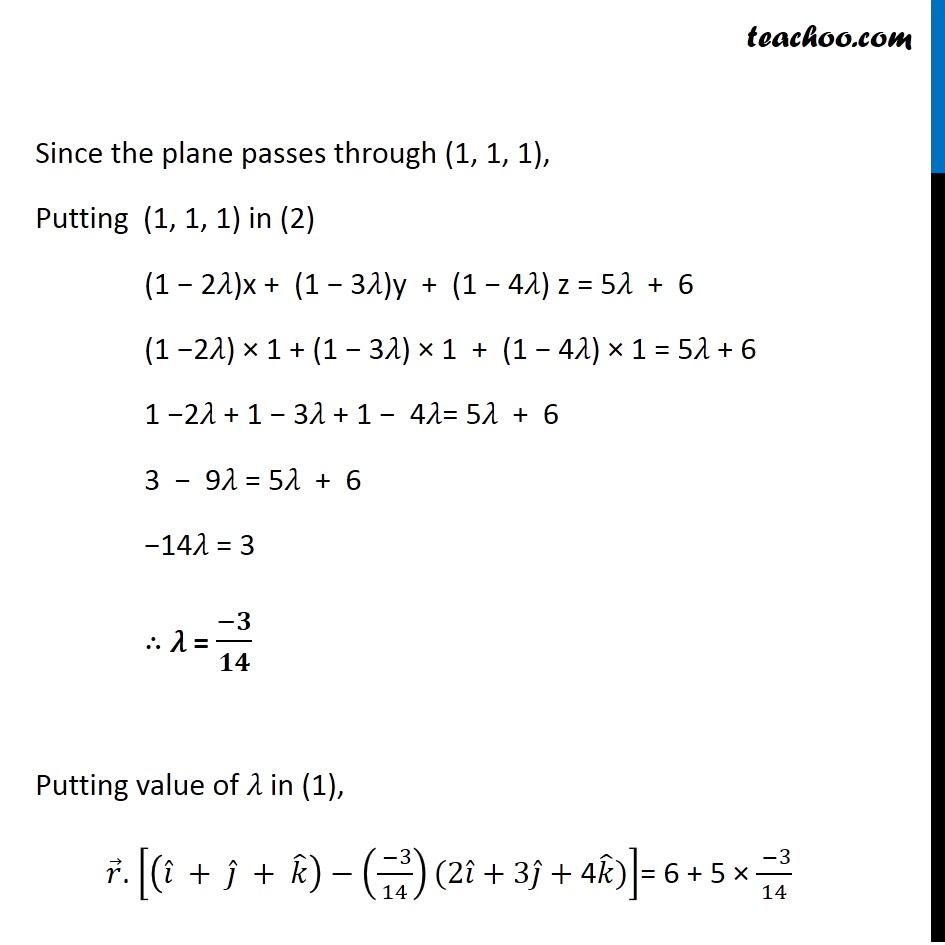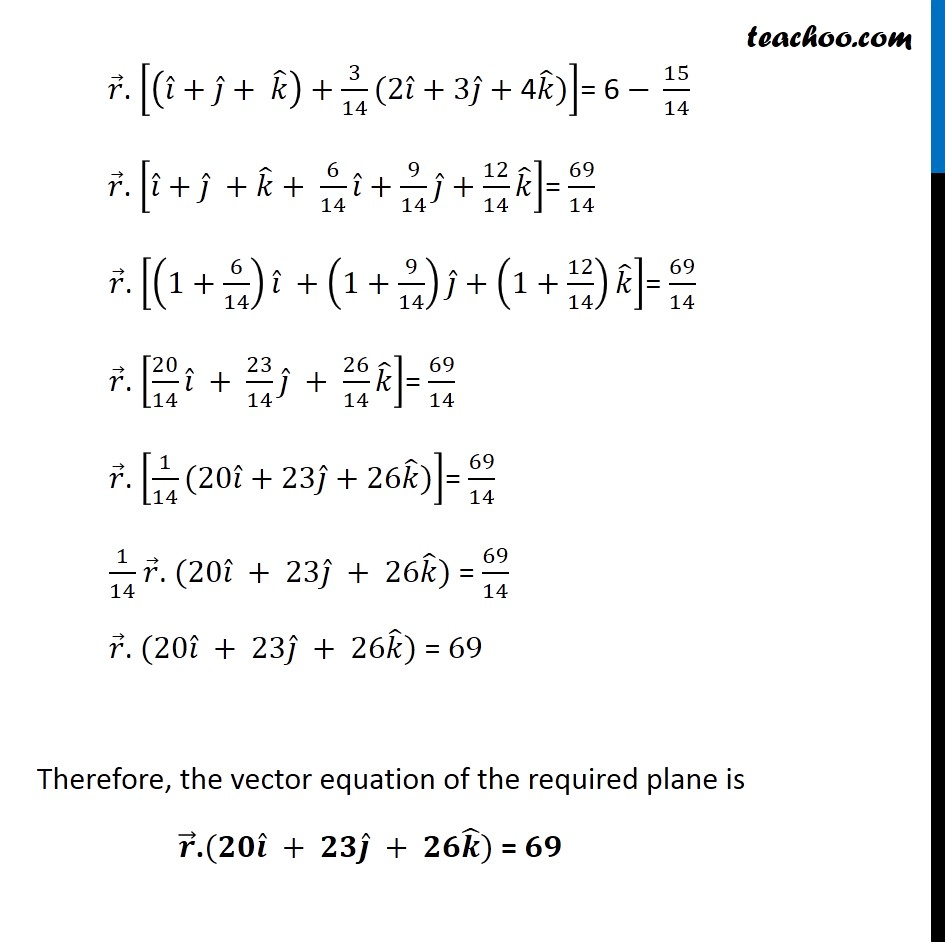Examples

Chapter 11 Class 12 Three Dimensional Geometry
Serial order wiseLearn in your speed, with individual attention - Teachoo Maths 1-on-1 Class

### Transcript

Question 10 Find the vector equation of the plane passing through the intersection of the planes 𝑟 ⃗ . (𝑖 ̂ + 𝑗 ̂ + 𝑘 ̂) = 6 and 𝑟 ⃗ . (2𝑖 ̂ + 3𝑗 ̂ + 4𝑘 ̂) = − 5, and the point (1, 1, 1).The vector equation of a plane passing through the intersection of planes 𝑟 ⃗. (𝑛1) ⃗ = d1 and 𝑟 ⃗. (𝑛2) ⃗ = d2 and also through the point (x1, y1, z1) is 𝒓 ⃗.((𝒏𝟏) ⃗ + 𝜆(𝒏𝟐) ⃗) = d1 + 𝜆d2 Given, the plane passes through 𝒓 ⃗.(𝒊 ̂ + 𝒋 ̂ + 𝒌 ̂) = 6 Comparing with 𝑟 ⃗.(𝑛1) ⃗ = d1, (𝒏𝟏) ⃗ = 𝒊 ̂ + 𝒋 ̂ + 𝒌 ̂ & d1 = 6 𝒓 ⃗.(2𝒊 ̂ + 3𝒋 ̂ + 4𝒌 ̂) = −5 –𝑟 ⃗.(2𝑖 ̂ + 3𝑗 ̂ + 4𝑘 ̂) = 5 𝑟 ⃗ .(− 2𝑖 ̂ − 3𝑗 ̂ − 4𝑘 ̂) = 5 Comparing with 𝑟 ⃗.(𝑛2) ⃗ = d2 (𝒏𝟐) ⃗ = − 2𝒊 ̂ − 3𝒋 ̂ − 4𝒌 ̂ & d2 = 5 Equation of plane is 𝑟 ⃗. [(𝑖 ̂+𝑗 ̂+𝑘 ̂ )+"𝜆" (−2𝑖 ̂−3𝑗 ̂−4𝑘 ̂)] = 6 + 𝜆5 𝒓 ⃗. [(𝒊 ̂" " +𝒋 ̂" " +𝒌 ̂ )−"𝜆" (𝟐𝒊 ̂+𝟑𝒋 ̂+𝟒𝒌 ̂)] = 6 + 5𝜆 Now to find 𝜆 , put 𝒓 ⃗ = x𝒊 ̂ + y𝒋 ̂ + z𝒌 ̂ (x𝑖 ̂ + y𝑗 ̂ + z𝑘 ̂). [(𝑖 ̂+𝑗 ̂+𝑘 ̂ )−"𝜆" (2𝑖 ̂+3𝑗 ̂+4𝑘 ̂)] = 5𝜆 + 6 (x𝑖 ̂ + y𝑗 ̂ + z𝑘 ̂).(𝑖 ̂+𝑗 ̂+𝑘 ̂ ) − 𝜆 (x𝑖 ̂ + y𝑗 ̂ + z𝑘 ̂).(2𝑖 ̂+3𝑗 ̂+4𝑘 ̂) = 5𝜆 + 6 (x × 1) + (y × 1) + (z × 1) − 𝜆[(𝑥×2)+(𝑦×3)+(𝑧×4)] = 5𝜆 + 6 x + y + z − 𝜆[2𝑥+3𝑦+4𝑧] = 5𝜆 + 6 x + y + z − 2𝜆𝑥 − 3𝜆y − 4𝜆z = 5𝜆 + 6 (1 − 2𝜆)x + (1 − 3𝜆)y + (1 − 4𝜆) z = 5𝜆 + 6 Since the plane passes through (1, 1, 1), Putting (1, 1, 1) in (2) (1 − 2𝜆)x + (1 − 3𝜆)y + (1 − 4𝜆) z = 5𝜆 + 6 (1 −2𝜆) × 1 + (1 − 3𝜆) × 1 + (1 − 4𝜆) × 1 = 5𝜆 + 6 1 −2𝜆 + 1 − 3𝜆 + 1 − 4𝜆= 5𝜆 + 6 3 − 9𝜆 = 5𝜆 + 6 −14𝜆 = 3 ∴ 𝜆 = (−𝟑)/𝟏𝟒 Putting value of 𝜆 in (1), 𝑟 ⃗. [(𝑖 ̂" " +" " 𝑗 ̂" " +" " 𝑘 ̂ )−(( −3)/14)(2𝑖 ̂+3𝑗 ̂+"4" 𝑘 ̂)]= 6 + 5 × ( −3)/14 𝑟 ⃗. [(𝑖 ̂+𝑗 ̂+" " 𝑘 ̂ )+3/14(2𝑖 ̂+3𝑗 ̂+"4" 𝑘 ̂)]= 6 − 15/14 𝑟 ⃗. [𝑖 ̂+𝑗 ̂" " +𝑘 ̂+ 6/14 𝑖 ̂+9/14 𝑗 ̂+12/14 𝑘 ̂ ]= 69/14 𝑟 ⃗. [(1+6/14) 𝑖 ̂ +(1+9/14) 𝑗 ̂+(1+12/14) 𝑘 ̂ ]= 69/14 𝑟 ⃗. [20/14 𝑖 ̂ + 23/14 𝑗 ̂ + 26/14 𝑘 ̂ ]= 69/14 𝑟 ⃗. [1/14(20𝑖 ̂+23𝑗 ̂+26𝑘 ̂)]= 69/14 1/14 𝑟 ⃗. (20𝑖 ̂ + 23𝑗 ̂ + 26𝑘 ̂) = 69/14 𝑟 ⃗. (20𝑖 ̂ + 23𝑗 ̂ + 26𝑘 ̂) = 69 Therefore, the vector equation of the required plane is 𝒓 ⃗.(𝟐𝟎𝒊 ̂ + 𝟐𝟑𝒋 ̂ + 𝟐𝟔𝒌 ̂) = 𝟔𝟗